# Lattice (Order Theory)

A lattice is a partially ordered set that is closed under binary joins and meets.

Let $$L$$ be a lattice. Then for all $$p,q,r \in L$$ the following properties are necessarily satisfied.

• Associativity of joins and meets: $$(p \vee q) \vee r = p \vee (q \vee r)$$, and $$(p \wedge q) \wedge r = p \wedge (q \wedge r)$$

• Commutativity of joins and meets: $$p \vee q = q \vee p$$ and $$p \wedge q = q \wedge p$$

• Idempotency of joins and and meets: $$p \vee p = p$$ and $$p \wedge p = p$$

• Absorption: $$p \vee (p \wedge q) = p$$ and $$p \wedge (p \vee q) = p$$

Lemma 1: Let $$P$$ be a poset, $$S \subseteq P$$, and $$p \in P$$. If both $$\bigvee S$$ and $$(\bigvee S) \vee p$$ exist then $$\bigvee (S \cup \{p\})$$ exists as well, and $$(\bigvee S) \vee p = \bigvee (S \cup \{p\})$$.

Proof: See the Join fu exercise in Join and meet: Exercises.

## Associativity

Let $$L$$ be a lattice and $$p,q,r,s \in L$$ such that $$s = p \vee (q \vee r)$$. We apply the above lemma, along with commutativity and closure of lattices under binary joins, to get

$$p \vee (q \vee r) = (q \vee r) \vee p = (\bigvee \{q, r\}) \vee p = \bigvee (\{q, r\} \cup \{p\}) =$$
$$\bigvee \{ q, r, p \} = \bigvee (\{p, q\} \cup \{r\}) = (\bigvee \{p, q\}) \vee r = (p \vee q) \vee r.$$

By duality, we also have the associativity of binary meets.

## Commutativity

Let $$L$$ be a lattice and $$p,q \in L$$. Then $$p \vee q = \bigvee \{ p, q \} = q \vee p$$. Binary joins are therefore commutative. By duality, binary meets are also commutative.

## Idempotency

Let $$L$$ be a lattice and $$p \in L$$. Then $$p \vee p = \bigvee \{ p \} = p$$. The property that for all $$p \in L$$, $$p \vee p = p$$ is called idempotency. By duality, we also have the idempotency of meets: for all $$p \in L$$, $$p \wedge p = p$$.

## Absorption

Since $$p \wedge q$$ is the greatest lower bound of $$\{p,q\}$$, $$p \wedge q \leq p$$. Because $$p \leq p$$ and $$(p \wedge q) \leq p$$, $$p$$ is an upper bound of $$\{p, p \wedge q\}$$, and so $$p \vee (p \wedge q) \leq p$$. On the other hand, $$p \vee (p \wedge q)$$ is the least upper bound of $$\{p, p \wedge q\}$$, and so $$p \leq p \vee (p \wedge q)$$. By anti-symmetry, $$p = p \vee (p \wedge q)$$.

<div><div>

## Closure under finite joins and meets

Let $$L$$ be a lattice and $$S = \{ s_1, ..., s_n \}$$ be some finite subset of $$L$$. Then an inductive argument shows that $$\bigvee S$$ exists.

Here again, we will need Lemma 1, stated in the proofs of the four lattice properties.

Our proof proceeds by induction on the cardinality of $$S$$.

The base case is $$\bigvee \{ s_1 \} = s_1 \in L$$. For the inductive step, we suppose that $$\bigvee \{s_1, ..., s_i \}$$ exists. Then, applying lemma 1, we have $$\bigvee \{s_1, ..., s_{i+1} \} = \bigvee \{s_1, ..., s_i \} \vee s_{i+1}$$. Applying our inductive hypothesis and closure under binary joins, we have $$\bigvee \{s_1, ..., s_i \} \vee s_{i+1}$$ exists. Lattices are therefore closed under all finite joins, not just binary ones. Dually, lattices are closed under all finite meets. <div><div>

## Basic positive examples

Here are two Hasse diagrams of posets which are lattices.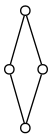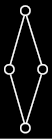comment:

dot source:

digraph G { node = 0.1, height = 0.1 edge = “none” a = “” b = “” c = “” d = “”

rankdir = BT; a → b a → c b → d c → d } <div>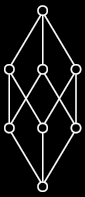comment:

dot source:

digraph G { node = 0.1, height = 0.1 edge = “none” a = “” b = “” c = “” d = “” e = “” f = “” g = “” h = “”

rankdir = BT; a → b a → c a → d b → e b → f c → e c → g d → f d → g e → h f → h g → h }

<div>

## Basic negative examples

Here are two Hasse diagrams of posets which are not lattices.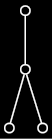comment:

digraph G { node = 0.1, height = 0.1 edge = “none” a = “” b = “” c = “” d = “” e = “” f = “” g = “” h = “”

rankdir = BT; a → b a → c a → d b → e b → f c → e c → g d → f d → g e → h f → h g → h }

<div>

In the above diagram, the two bottom elements have no common lower bounds. Therefore they have no meet, and so the depicted poset is not a lattice. However, it should be easy to verify that this poset is closed under binary joins.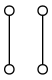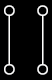comment:

dot source:

digraph G { node = 0.1, height = 0.1 edge = “none” a = “” b = “” c = “” d = “”

rankdir = BT; a → b c → d }

<div>

The Hasse diagram of this poset has two connected components. No element from the left component will have a meet or a join with any element from the right component. The depicted poset is therefore not a lattice.

## The connecting lemma

The connecting lemma states that for any lattice $$L$$ and $$p,q \in L$$, $$p \vee q = p \Leftrightarrow q \leq p$$ and dually, $$p \wedge q = p \Leftrightarrow q \geq p$$. This simple but important lemma is so named because it establishes a connection between the lattice’s join operator and its underlying poset order.

We prove $$p \vee q = p \Leftrightarrow q \leq p$$; the other part follows from duality. If $$p \vee q = p$$, then $$p$$ is an upper bound of both $$p$$ and $$q$$, and so $$q \leq p$$. Going the other direction, suppose $$q \leq p$$. Since $$p$$ is and upper bound of itself by reflexivity, it then follows that $$p$$ is an upper bound of $$\{p, q\}$$. There cannot be a lesser upper bound of $$\{p, q\}$$ because it would not be an upper bound of $$p$$. Hence, $$p \vee q = p$$.

<div><div>

## Lattices as algebraic structures

It’s also possible to formulate lattices as algebraic structures $$\langle L, \vee, \wedge \rangle$$ satisfying the associativity, commutativity, idempotency, and absorption laws described above. A poset $$\langle L, \leq \rangle$$ can then be defined such that for $$p, q \in L$$, $$p \leq q$$ whenever $$p \vee q = q$$. It can be shown that this poset is closed under binary meets and joins, and that these meets and joins are equal to the corresponding meets and joins of the algebraic lattice.